A# isotope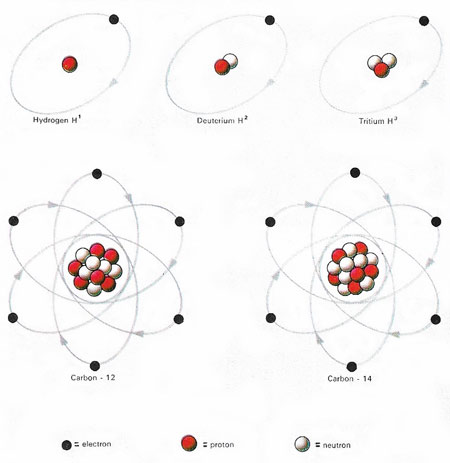Fig 1. The isotopes of an element all contain the same number of protons but differ in neuron number. The hydrogen atom has one proton. The common form has no neurons, the rarer form, deuterium, has one; tritium, with two neurons, is radioactive. The stable isotope of carbon, carbon-12, has six protons and six neurons; carbon -14, with eight neurons, is radioactive. The ratio of these two in once-living material helps determine its age.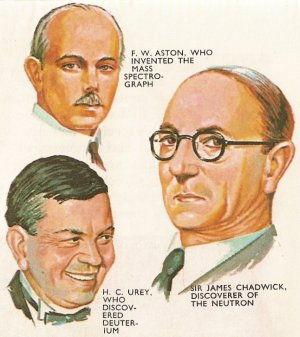Fig 2. Pioneers of the physics of isotopes: Aston, Chadwick, and Urey.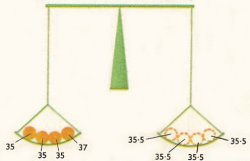Fig 3. Ordinary chlorine consists of 3 atoms of 35Cl to 1 atom of 37Cl so the measured atomic weight (i.e. the average atomic weight) = 35.5.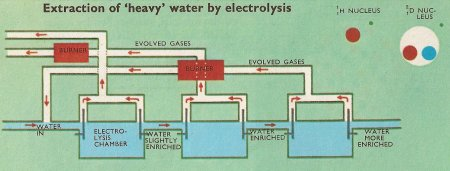Fig 4. Water consists of a mixture of the oxides of the two isotopes 1H and 2D. The 2D atom is twice as heavy as the 1H atom and is evolved at the cathode at a lower rate than the 1H atom. The residue is thus enriched in 2D atoms. In the practical process, water containing a little caustic soda is fed into the first electrolyzing chamber. Most of it is here electrolyzed before being passed into the second chamber. The gases evolved are burnt and passed back into the system two stages back to reduce losses of deuterium. By pumping water continuously through several stages, heavy water of high purity is obtained.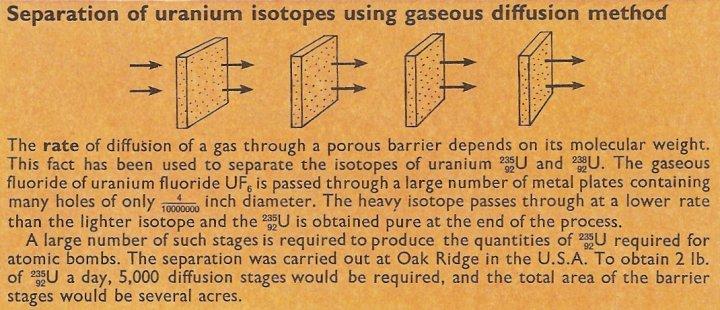Fig 5. Uranium isotope separation by diffusion.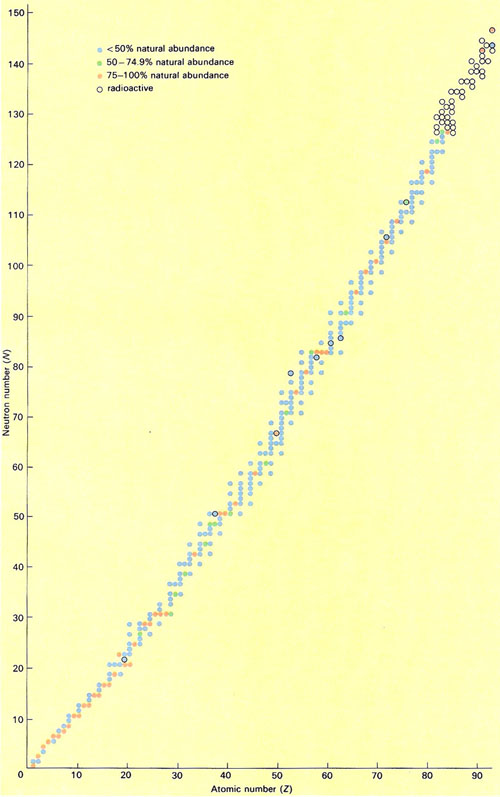Fig 6. The isotopes found in measurable quantities in nature, together with the members of the three naturally-occurring radioactive decay series. Neutron number (N) is plotted against atomic number (Z).

Isotopes are atoms of the same element which differ in the number of neutrons they contain (Fig 1). For example, helium-3 (3He), with two protons and one neutron in each nucleus, and helium-4 (4He), with two protons and two neutrons, are two different isotopes of helium. Nearly all elements found in nature are mixtures of several different isotopes. Although the chemical properties of isotopes of the same element are the same, the physical properties differ. The natural proportions of the isotopes are expressed in the form of an abundance ratio.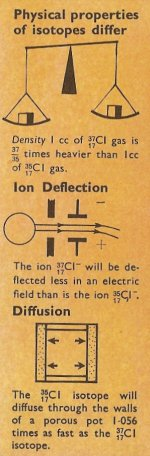## Discovery of isotopes

By the end of the eighteenth century many facts were known about the way that chemical elements combine. For example, it was known that if 1 g of element A reacts with 10 g of element B, then 2 g of A will react with 20 g of B. This was explained, in 1803, by the chemist John Dalton, who suggested that all matter is composed of tiny particles, called atoms. In Dalton's theory, all the atoms of the same element had the same weight, and elements differed from each other because they were composed of atoms of different weights.

From measurements of the amounts of different substances which took part in chemical reactions, it was possible to measure the atomic weight (also known as the relative atomic mass) of these elements. The atomic weight of an element is the weight of an atom of the element compared with that of a hydrogen atom.

In 1815, William Prout noticed that most atomic weights were almost exactly whole numbers, so that the weight of each atom was almost exactly a multiple of that of a single hydrogen atom. For example, the nitrogen atom is 14 times, and the oxygen atom 16 times, as heavy as the hydrogen atom. This led him to suggest that all atoms were composed of hydrogen atoms. However, when more accurate measurements of atomic weights were made, it was found that they were not exactly whole numbers. Chlorine, for example, was found to have an atomic weight of 35.5. This brought about the downfall of Prout's hypothesis, and it was not until the atomic scientists of the 20th century made their discoveries about the structures of individual atoms that a proper explanation of the values of atomic weights could be found.

In 1897, J. J. Thomson discovered the electron, and in 1911 Ernest Rutherford discovered that the atom consists of a small positively charged core, called the nucleus, surrounded by a number of negatively charged electrons. The positive charge on the nucleus is exactly equal to the total of negative charges carried by the electrons.

The number of units of positive charge on the nucleus of the atom is called the atomic number of the atom, and in 1913 H. G. J. Mosely found the atomic numbers of several elements.

It is the number and arrangement of electrons in the atom which determines its chemical behavior. The atomic weights merely determine the amounts of each element which take part in a chemical reaction.

It is therefore possible to have two atoms of the same element of different atomic weight, but which have identical chemical properties because the atomic numbers (and therefore the numbers of electrons in the atoms) are the same. Such atoms are called isotopes. Francis W. Aston, in 1919, using a mass spectrograph, was able to separate isotopes of different elements. In the mass spectrograph it is possible to separate atoms of different atomic weights, and to determine how much of each isotope is present.

By making such measurements, it has been possible to find out how much of each isotope exists in each element, and to explain the values of atomic weights of the elements. For example, chlorine has two naturally occurring isotopes of atomic weights 35 and 37, and it has been found that there is almost three times as much of the lighter isotope as there is of the heavier one. This leads to an average atomic weight of (3 × 35 + 1 × 37)/4 = 35.5.

The atomic weights of the isotopes of the elements are very nearly whole numbers, and the reason for this became apparent when James Chadwick, in 1932, discovered the neutron (Fig 2). The neutron is an uncharged particle of mass one. It became clear that the atomic nucleus was composed of protons (particles of mass one carrying a single positive charge) and neutrons. For example, the nucleus of helium, which has an atomic weight of 4 and an atomic number of 2, consist of 2 neutrons and 2 protons. The nucleus of the chlorine isotope atomic weight 35 consists of 17 protons and 18 neutrons, whilst the chlorine isotope atomic weight 37 consists of 17 protons and 20 neutrons. The atomic number of both isotopes is 17.

## Separation of isotopes

Whilst the chemical properties of an element, as determined by the number and arrangement in shells of the electrons, are the same, the physical properties differ. It is this difference in physical properties which enables separation of the isotope to be carried out. For example, the most obvious difference between isotopes of an element is that of mass. This leads to differences in the rates of diffusion of the different gaseous isotopes of an element through a porous membrane, and to different rates of evaporation in liquid elements. Both of these properties have been used to separate isotopes.

Another example is the electrolytic separation of the two naturally occurring isotopes of hydrogen. Hydrogen consists of a mixture of two isotopes; the nucleus of the lighter one (atomic weight 1) consists of a single proton. The nucleus of the heavier isotope (atomic weight 2) consists of a neutron and a proton, and is called the deuteron. The atom of the heavier isotope is called deuterium and is denoted in chemical equations by writing a "D". Just as hydrogen forms the oxide H2O (ordinary water), so deuterium forms the oxide D2O (heavy water). The weight of a molecule of heavy water is (16 + 2 + 2)/(16 + 1 + 1) = 20/18 times as great as that of a molecule of ordinary water.

In ordinary water there are 660 ordinary H2O molecules to every heavy water molecule. When water containing sodium hydroxide is electrolyzed, oxygen is evolved at the anode (positive electrode). Both hydrogen and deuterium are evolved at the cathode (negative electrode). Because the deuterium molecules are twice as heavy as the hydrogen molecules, they are evolved at a lower rate than the hydrogen molecules, and tend to be left behind, in solution. Enrichment of the solution with the heavy isotope therefore results, but it is only a slight enrichment, because the proportion of heavy atoms present in the solution is small, and some of these atoms are evolved with the light ones. The process has therefore to be repeated many times with successive additions of water, followed by electrolysis, until the required concentration is obtained. To obtain 1 g of 99% pure heavy water, 100,000 g of ordinary water has to electrolyzed.

Similar difficulties are encountered in the other methods of separating isotopes. For example, in the diffusion method of separation, passage through a plate containing tiny holes will separate gaseous compounds of isotopes of the element uranium. In practice, to obtain 99% purity of the U235, 5,000 such plates, through which the gas diffuses in succession, would be required.

So while there are many ways of separating isotopes – others include distillation (formerly used to produce heavy water), centrifuging, and laser methods – none is easy in practice, and it is not surprising that the discovery of isotopes had to wait for the work of twentieth century physicists and chemists.